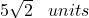## 10) Find the distance between (2, 3) and (-3, -2) A) 2 B) 5 C) 50 = 5 2 D) 25

Question

10) Find the distance between (2, 3) and (-3, -2) A) 2 B) 5 C) 50 = 5 2 D) 25

in progress 0
2 weeks 2021-07-22T23:14:25+00:00 1 Answers 2 views 0

##Step-by-step explanation:

The distance between two points can be found by using the formulawhere

(x1 , y1) and (x2 , y2) are the points

From the question the points are

(2, 3) and (-3, -2)

The distance between them isWe have the final answer asHope this helps you